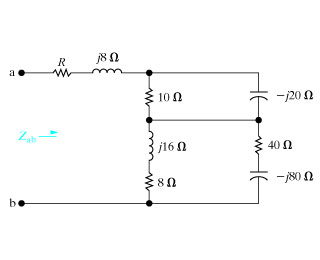Create an Account

Home / Questions / Item 2 Find the impedance Zab in the circuit seen in the figure Figure 1 Suppose that R ...

Item 2 Find the impedance Zab in the circuit seen in the figure Figure 1 Suppose that R 15 Part A Express Zab in polar form Enter your answer using polar notation Express argument in

Item 2

Find the impedance Zab in the circuit seen in the figure.(Figure 1) . Suppose that R = 15? .

Part A

Express Zab in polar form.

Zab =     ?

Part B

Express Zab in rectangular form.

Zab =     ?Subscribe To Get Solution